# Modelling the Triple-Dichotomy

### Introduction

 I present here an attempt to elucidate the relationship between the analytic/synthetic and the necessary/contingent dichotomies making use of formal models. The material is therefore a kind of methodological experiment, in the application of formal methods to philosophical problems.

### Preliminaries

 Preconditions for Deductive Reasoning The history of logic over the last century has shown how easy it is to construct formal logical systems which are inconsistent. Nevertheless, the logical foundations on which mathematics is based have been extremely stable now for at least half a century, so we can reasonably say that the preconditions for reliable deductive reasoning are well enough understood. These lessons have however had marginal effect on the way analytic philosophy is conducted and the potential benefits of adopting mathematical standards of rigour in philosophy are not widely appreciated. Set Theory good for Philosophy This page covers an example intended to explore the application of "mathematical" modelling techniques in philosophy. I am presuming here (and will be arguing elsewhere) that set theory is not specifically mathematical in character, but provides a general purpose logical and abstract ontological framework applicable to all demonstrative reasoning. A major deficit in typical philosophical arguments is the the failure to identify the subject matter of the discourse. Set theory provides the techniques necessary to remedy this deficit. Formality not Necessary Possibly one of the reasons why there is so little use of rigorous logical techniques in philosophy is the daunting prospect of dealing with complex formal arguments of the kind presented in Principia Mathematica. Mathematicians have been equally disinclined to working at this level of detail and have found acceptable and effective compromises which deliver most of the benefits with a great deal less of the pain. The standard of mathematical demonstration in the twentieth century has been formalisability in a classical set theory (such as ZFC), but mathematicians are happy to be persuaded that this standard has been met without actually being shown a formal proof. The Triple-Dichotomy The example presented here concerns the fundamental triple-dichotomy, analytic/synthetic, necessary/contingent, a priori/a posteriori. The idea is to provide a simple set-theoretic model of the relevant domain, to offer definitions of these concepts using that model, and to derive some elementary results about the relationships between the concepts. Apart from exploring the applicability of set theory in analytic philosophy it is intended to examine the minimal conditions for these dichotomies to be well defined as a part of an assessment of the significance of various skeptical arguments in relation to these concepts.

### The Model

This model actually only covers two of the dichotomies and is intended to suggest definitions under which the coincidence of the dichotomies is itself analytic. I can see no useful way to model the a priori/a posteriori dichotomy since these concepts I suggest should be considered as having no descriptive content, but rather as expressing or flowing from an attitude or policy on what kinds of justification should be expected for different kinds of conjecture. The policy I adopt and recommend is that a priori justification should be required and accepted if and only if the conjecture is analytic, and that an a posteriori justification is appropriate for synthetic conjectures. The adoption of this policy in no way contributes to the meaning of the concepts involved, though in the absence of a policy there is no way to determine whether any specific conjecture demands a priori or a posteriori justification.

#### Use of Symbols

In the following model I first experimented with the "cascading style sheets", a feature incompletely implemented in recent bowsers which permits fonts to be selected. Having established that works in too few browsers to make sense I have now switched back to my old method of using tiny gifs for the special characters, which works fine.

The small set of special symbols used are shown in the following table:

 forallx:A.P asserts the formua P (which may contain free occurrences of the variable x) for all value of x in A exists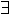x:A.P asserts the formua P (which may contain free occurrences of the variable x) for some value of x in A notP the logical negation of P member a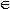B or a:B a is a member the set B function space A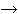B the set whose members are the functions with domain A and co-domain B cartesian product A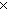B the set whose members are all the ordered pairs of which the first element is a member of A and the second a member of B
 A descriptive language is a 6-tuple consisting of: A set of sentences S. A set of contexts C. A set of possible worlds W. A set of proposions P. A semantic map m(SC)P. A propositional evaluation map v(PW){T,F,U}. Where T, F, and U are three arbitrary but distinct sets which represent the truth values true, false and undefined.
 A statement is: an ordered pair s:S, c:C An ordered pair consisting of a sentence s and a context c. A proposition p is necessarily true iff:w:W. v(p,w) = T For every possible world w, p is true in w. A proposition p is necessarily false iff:w:W. v(p,w) = F For every possible world w, p is false in w. A proposition p is necessary iff:t:{T,F,U}.w:W. v(p,w) = t There exists some truth value t which is the truth value of p in every possible world w. A proposition p is contingent if:w1,w2:W.(v(p,w1) = v(p,w2)) There exist two possible worlds w1 and w2 such that the truth value of p in w1 differs from its value in w2 A statement s is necessary (contingent) iff: the proposition m(s) is necessary (contingent) A statement s is analytic iff:t:{T,F,U}.w:W.v(m(s),w)=t There exists some truth value t which is the truth value of s in every possible world w.

I might now make a show of demonstrating that the two dichotomies are coincident. However, the differences between the proferred definitions of analytic and necessary are too small for that to be very impressive. Once the underlying model is in place it is already pretty clear that the definition of analytic will be the same as that of necessary. This is because the model makes explicit the features on which the truth of a statement depends and it is then obvious that to state that one of these features suffices to determine the truth value is just the same as stating that the truth value is independent of the other. There seems to be no way to say that meaning suffices without quantifying over the possible worlds and asserting that the truth value does not change.

The entire value of the model therefore depends upon its power to make clear the meaning of the concepts involved, and I now therefore consider whether in that respect the model is a good model.

I have attempted to make the model as general as possible, so that the concepts involved will be meaningful for as broad a range of languages as possible. For this reason I have not assumed that a sentence will in all contexts and all possible worlds have a truth value. This of course does not preclude languages in which this is the case. It does introduce some additional complexity into the definitions of analyticity and necessity, the obvious generalisation resulting in there being the strange category of analytically undefined statements.

The second feature intended to provide greater generality is the use of propositions as intermediaries in the semantic/evaluation mappings. At first glance this might be thought to restrict the applicability of the model by excluding those languages for which the notion of proposition is for some reason inapplicable. However, sufficiently little is said about the nature of propositions that subject to minimal criteria about the semantic well definedness of the language there will be at least two candidates for the role of proposition required by this model.

To understand this it is beneficial to imagine how the model might be if propositions were not involved. In the worst case we must assume that the truth value of statements in any possible world is either true or false or else undefined. This could be captured by a single relation, or a function f:(S x C x W){T,F,U}.

Given such a definition of the semantics of a language we can construct two canonical propositional semantics. In the first a proposition is a statement in context and the semantic map (m) is the identity function. In the second a proposition is a map from possible worlds to truth values. The semantic map is defined by:

m(s,c)(w) = f(s,c,w)
(i.e. m(s,c) is a function which, when applied to a possible world, yields a truth value). The evaluation function (v) applies the "proposition" (m(s,c)) to a possible world to obtain a truth value, i.e.:
v(p,w) = p(w)

The greater generality of the propositional model is simply in failing to prejudice what value we identify as the meaning of the statement. This is desirable since neither of the canonical renditions is very satisfactory as an account of meaning. If the canonical constructions of propositions are considered as meanings, then in the first case meaning is simply identified with the statement (in context), and the possibility that two distinct statements could have the same meaning is excluded. In this case "the meaning" has too much information in it. In the second case the meaning contains perhaps too little information. It results, for example, in all true analytic statements having the same meaning.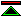©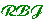created 1997-6-1 modified 2001-10-17